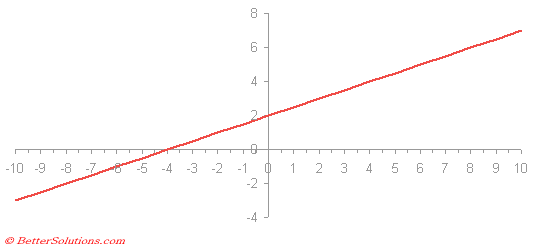# Linear Equation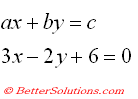### Example

Lets suppose that we have a linear equation and we want to find the solution.
A linear equation is an equation that
One way to find the root is to use Goal Seek.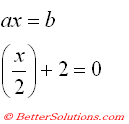### Create the Table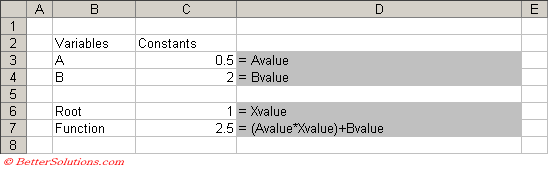### Using Goal Seek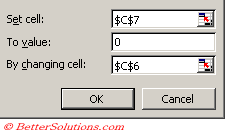### Checking the Result